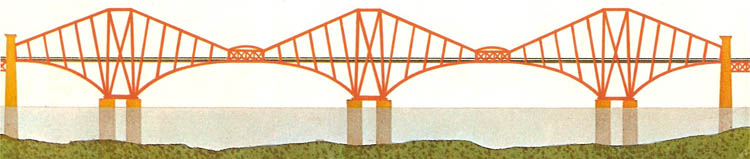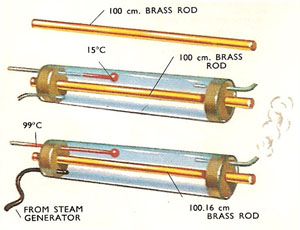A# linear expansionThe Forth railway bridge has an overall length of 2,529 m (about 1½ miles). It is made of steel (coefficient of linear expansion = 0.000012 per °C). If the greatest difference in temperature between the coldest and hottest days is 35°C the length of the bridge can change by 2529 × 0.000012 × 35 = 1.06 m. In fact, provision is made for an expansion of 1.8 m.A brass rod exactly 100 cm long at 15°C is heated in a steam jacket and the resulting increase in length is found to be 0.16 cm. If the temperature of the steam is 99°C, what is the coefficient of linear expansion of brass?

Increase in length = Lo × α × t = 0.16 cm,
original length (Lo) = 100 cm,
rise in temperature (t) = 99 - 15 = 84°C.
Hence, 0.16 = 100 × α × 84,
so that α = 0.000019 per °C.

A steel bridge is 100 m long when the temperature is 2°C. What will be its length when the temperature is 27°C? Unless provision is made for the increase in length linear expansion, as it is called – the bridge could buckle disastrously on a hot day. So before the bridge is built, engineers must be able to calculate the increase in length. In this example, it is just over 2½ cm.

The increase in length depends upon three things. First, as might be expected, it is proportional to the original length of the structure. For example, a steel bridge 100 m long will expand 10 times as much as a steel rail 10 meters long when both are heated equally. Secondly, the increase in length is proportional (or practically so) to the increase in temperature which the structure undergoes. For example, if a particular specimen expands by 1 mm when its temperature is raised through 25°C, it will expand by 4 mm when its temperature is raised through 11°C. Thirdly, the increase in length depends on the material from which the structure is made.

As usual, when a quantity is proportional to a number of factors it is also proportional to the factors multiplied together (i.e., the product of the factors). Hence the increase in length of a specimen is proportional to the original length × the increase in temperature. To find the actual increase in length, however, the product is multiplied by a number, which depends on the material, called the coefficient of linear expansion. The increase in length then equals the original length × coefficient of linear expansion × increase in temperature. This equation can be written as:

Increase in length = Lo × α × t

where Lo is the original length, α is the coefficient of linear expansion, and t is the increase in temperature.

With the aid of this equation it is easy to solve the bridge problem we posed earlier. The original length (Lo of the bridge is 100 m, the coefficient of linear expansion of steel is 0.000012 per °C, and the rise in temperature (t) is 27 - 2 = 25°C. The increase in length is therefore 100 × 0.000012 × 25 m = 0.03 m = 3 cm.

The same equation can be used to find the decrease in length of a solid when the temperature decreases. What will be the length of the bridge when the temperature drops to minus 13°C (a temperature decrease of 15°C)? The answer is 100 × 0.000012 × 15 = 0.018 m = 1.8 cm, and the length of the bridge at -13°C is 100 - 0.018 m = 99.982 m.

## Linear, superficial, and cubical expansion

Often the equation for linear expansion is given as:

Lt = Lo(1 + αt)

where Lt is the new length after expansion, α the coefficient of linear expansion, and t the rise of temperature.

A similar equation for calculating the increase in area (superficial expansion) is At = Ao(1 + βt), where At is the new area after expansion, Ao the original area, and β the coefficient of superficial expansion. β is equal to twice the coefficient of linear expansion, i.e., β = 2α.

The equation for calculating the increase in volume (cubical expansion) is Vt = Vo(1 + γt), where Vt is the new volume after expansion, Vo the original volume, and γ the coefficient of cubical expansion. γ is equal to three times the coefficient of linear expansion, i.e., γ = 3α.